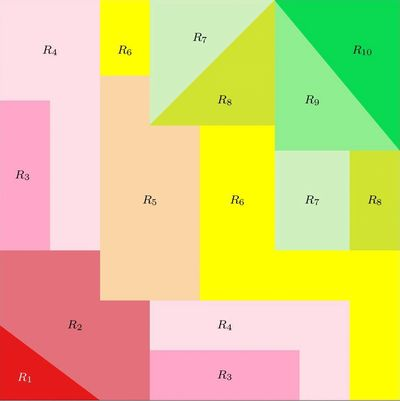#### MIGO: Machine Intelligence via Graph Optimization

MIGO belongs to a class of Artificial Intelligence called Machine Learning (ML). It uses a semi-supervised learning algorithm where the system is given “samples” used for training. A sample is an [input,target] pair and the objective is to minimize the miss-classification of the targets.

MIGO uses a type of ML algorithm called Classification Trees, but here is where any similarity to any other tree-based algorithm ends. Compared to other traditional ML algorithms (such as Neural Networks or Support Vector Machines), Classification Trees have the unique property that, however complex the derivation algorithm may be, the solutions themselves are easily understandable and simple to explain, giving insight, sensitivity and therefore trust into the answer. Inputs to the Classification Tree are called “factors” and the set of inputs used to create a single tree is called the “factor-set”.  For MIGO, the factor-sets are derived using a branch of mathematics called Graph Theory.

MIGO creates a collection of Classification Trees, called a “forest”. The outputs from each tree of the forest are then combined using an evolutionary algorithm, to produce the final output of the system.

The MIGO Classification Trees are created by proprietary, graph optimization algorithms which guarantee (with mathematical proof) that the produced trees are optimal. In addition, the generated trees possess another unique and superior property: namely, they incorporate both orthogonal and skewed cuts of the sample space. The trees also have a deep-learning characteristic which is unique to MIGO. The resulting Classification Trees have superior performance to any other existing system.

For white-papers or questions regarding the MIGO system, please email us at info@rekiki.com